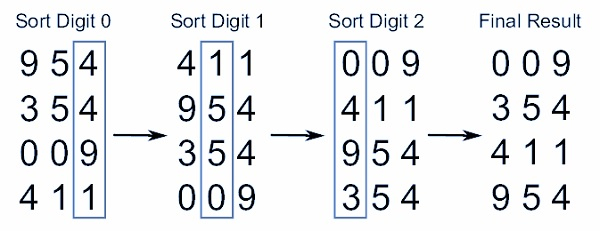# C Program for Radix Sort

A sorting algorithm is an algorithm that puts components of a listing in a certain order. The most-used orders are numerical order and lexicographic order.

The Radix sort is a non-comparative sorting algorithm. The Radix sort algorithm is the most preferred algorithm for the unsorted list.

It sorts the elements by initially grouping the individual digits of the same place value. The idea of Radix Sort is to do digit by digit sort starting from least significant digit(LSD) to the most significant digit(MSD), according to their increasing/decreasing order. Radix sort is a small method that is used several times when alphabetizing an oversized list of names. Specifically, the list of names is initially sorted according to the first letter of every name, that is, the names are organized in twenty-six categories.

Let us review the following illustration to understand clearly about the working of the radix sort algorithm. Clearly, the number of pass/iteration depends on the size of the highest individual number.In the above example, the primary column is input. The remaining columns show the list after successive sorts on increasingly significant digits position.

Complexity Analysis of Radix Sort O(m.n).

However, if we glance at these 2 values, the size of the keys is comparatively small in comparison to the number of keys. as an example, if we've six-digit keys, we might have 1,000,000 totally different records.

Here, we tend to see that the size of the keys isn't important, and this algorithm is of linear quality O(n)

## Algorithm

Radix_sort (list, n)
shift = 1
for loop = 1 to keysize do
for entry = 1 to n do
bucketnumber = (list[entry].key / shift) mod 10
append (bucket[bucketnumber], list[entry])
list = combinebuckets()
shift = shift * 10

## Example

This is a C Program to implement Radix Sort.

Live Demo

#include<stdio.h>
int get_max (int a[], int n){
int max = a;
for (int i = 1; i < n; i++)
if (a[i] > max)
max = a[i];
return max;
}
void radix_sort (int a[], int n){
int bucket, bucket_cnt;
int i, j, k, r, NOP = 0, divisor = 1, lar, pass;
lar = get_max (a, n);
while (lar > 0){
NOP++;
lar /= 10;
}
for (pass = 0; pass < NOP; pass++){
for (i = 0; i < 10; i++){
bucket_cnt[i] = 0;
}
for (i = 0; i < n; i++){
r = (a[i] / divisor) % 10;
bucket[r][bucket_cnt[r]] = a[i];
bucket_cnt[r] += 1;
}
i = 0;
for (k = 0; k < 10; k++){
for (j = 0; j < bucket_cnt[k]; j++){
a[i] = bucket[k][j];
i++;
}
}
divisor *= 10;
printf ("After pass %d : ", pass + 1);
for (i = 0; i < n; i++)
printf ("%d ", a[i]);
printf ("");
}
}
int main (){
int i, n, a;
printf ("Enter the number of items to be sorted: ");
scanf ("%d", &n);
printf ("Enter items: ");
for (i = 0; i < n; i++){
scanf ("%d", &a[i]);
}
printf ("Sorted items : ");
for (i = 0; i < n; i++)
printf ("%d ", a[i]);
printf ("");
return 0;
}

## Output

Enter number of items to be sorted 6
Enter items:567 789 121 212 563 562
After pass 1 : 121 212 562 563 567 789
After pass 2 : 212 121 562 563 567 789
After pass 3 : 121 212 562 563 567 789
Sorted items : 121 212 562 563 567 789